# Structure

## Structure | Why we should learn Structure

In this article, I am going to discuss the basics of Structure which is required to learn data structure and algorithm. Please read our previous article where we discussed the basics of Arrays.

##### What is Structure in C?

The structure can be defined as a collection of related data members under one name. Those data members may be of similar type or may be of dissimilar type. So usually it is defined as a collection of dissimilar data items under one name.

Structure in C is used for defining user-defined data types. Apart from the primitive data type we have in any programming language, for example in C language, we have primitive data types such as integer, float, double, etc. Using these primitive data types, we can also define our own data type depending on our own requirements. And this possible in C because of Structure.

In this course, I am going to use Structure more frequently. In this article, we will learn how to define the structure and what does it mean by the size of a structure like how much memory it consumes? Then we will discuss how to declare a structure and how to access the members of a structure?

##### Example to understand Structure:

Let us take an example of a rectangle. A rectangular is a figure having two properties i.e. length and breadth as shown in the below image.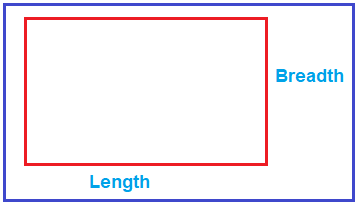So, a rectangle will have length and breadth, which means these two things (length and breadth) together defines a rectangle. So, in your programs, if you need something like a rectangle then you need to define a structure for it because a rectangle is not defined by just one value rather than is defined by a set of related values i.e. length and breadth. So, you can group them together under one name and define it as a structure.

For example, you need to define a structure for a rectangle as shown below.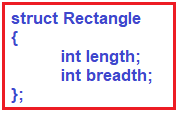As shown in the above image, we are creating struct Rectangle, inside this, we have integer type length, an integer type breadth. Now, this struct rectangle is having two members (length and breadth).

We are taking length and breadth as an integer type, but you can also take them as float or double or any other type depending on your business requirement. We are assuming that these are simple values which are integral type. So, the structure rectangle is defined by its length and breadth or we can say these are two data members together define a rectangle. This is the definition of a structure

##### How much memory this rectangle will be consuming?

It is having two integer members. Integer takes 2 bytes or it may take 4 bytes depending upon the Operating Systems. But let’s assume it takes 2 bytes. So, it will take a total of 4 bytes (2 bytes for length plus 2 bytes for breadth) of memory.

So, this rectangle structure is taking 4 bytes of memory. Right now, it is not consuming any memory because it is just a definition. So, if we create a variable of this type, then it will be occupying that much of memory. We have learned how to know its size.

Note: The size of a structure is the total amount of memory consumed by all its member.

##### How to declare a variable of type structure?

Please have a look at the below code.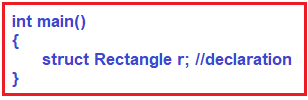As you can see in the above code, inside the main() method we declare a variable. So, the method of structure variable declaration is writing struct as a keyword then gives the structure name i.e. Rectangle, and followed by the variable name i.e. in this case ‘r’. This is the declaration of a structure variable. Now, this r will occupy the memory space and this will be created in the memory.

We can also declare and initialize at the same time as shown below.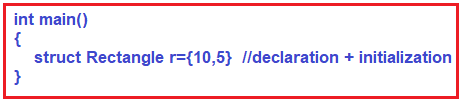As you can see in the above image, the struct variable ‘r’ is created with the values 10 and 5. Here the value 10 is assigned to length and the value 5 is assigned to breadth is 5.

##### Where this r variable is created inside the Main memory?

The variable r is going to be created inside the stack frame of the main memory as shown in the below image.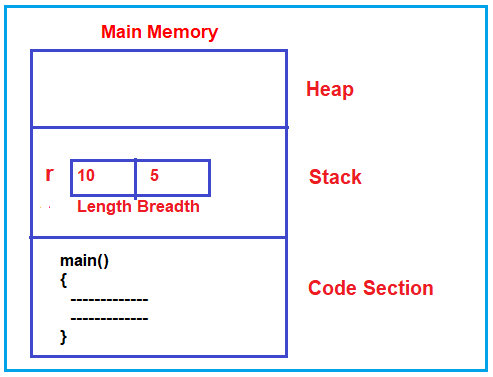##### How to access the member of the structure?

Suppose, you want to access the length of the structure i.e. you want to modify the length value to 15. For accessing the member of a structure, we need to use the structure variable name and the dot operator and followed by the structure member name. The following code shows how to modify the length of the structure.

r.length=5

##### What is the operator used for accessing the member?

The dot (.) operator is used for accessing a member of a structure. So, if you want to read and write the members of a structure then you need to use the dot operator. Let us write the complete example which will calculate the area of a rectangle.

```#include <stdio.h>
struct Rectangle
{
int length;
};

int main()
{
struct Rectangle r = { 10, 5 };
r.length = 20;